# CHOOSE Function in Excel

• Last Updated : 11 Aug, 2021

The CHOOSE function is technically part of Excel’s lookup function and can be incredibly useful. The CHOOSE function returns a value from a list using an index.

Syntax:

Attention reader! Don’t stop learning now. If you are an Excel beginner (or an intermediate) and want to learn Excel, Geeksforgeeks brings the perfect course for you to start, Diving Into Excel

`=CHOOSE(index_num, value1, [value2], ...)`

Parameter:

• Index_num: The value we want to choose. The number must be between 1 and 254.
• Value1: The first value from which to choose.
• Value2 (Optional): The second value from which to choose.

Let’s begin with the examples.

Example 1: To get our Value, follow the below steps: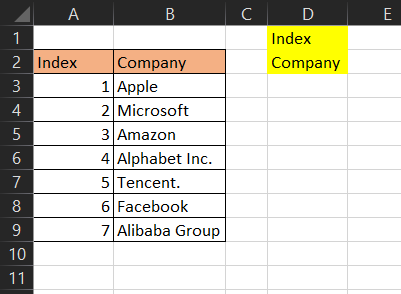Now, if we want to get the value of any number (index) in E1. Let us follow the next step

Step 2: We will enter =CHOOSE(E1,B3,B4,B5,B6,B7,B8,B9) in E2 cell.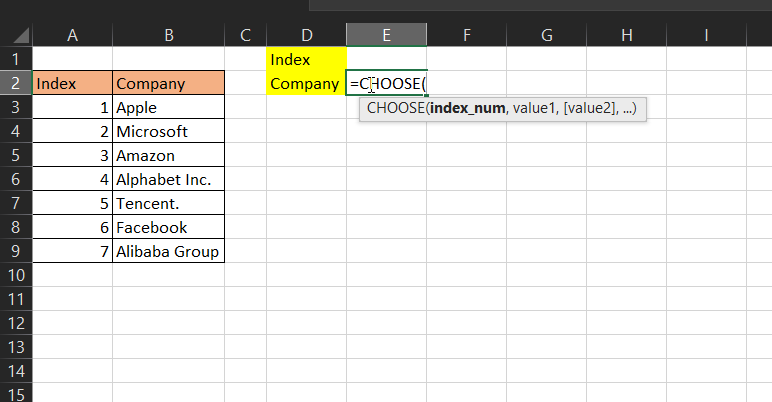Here we said Excel should return our index (E1) from our list; B3,B4,B5,B6,B7,B8,B9.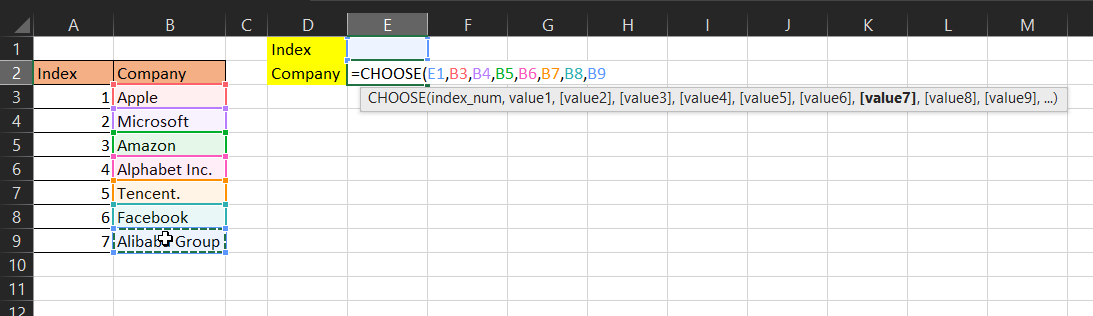This will return a #value! error because there is no number provided in E1.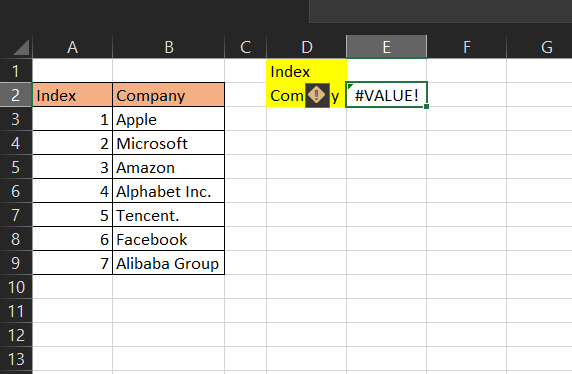Let’s put 1 in E1. The CHOOSE function returns Apple because Apple is the value with index 1 on your list.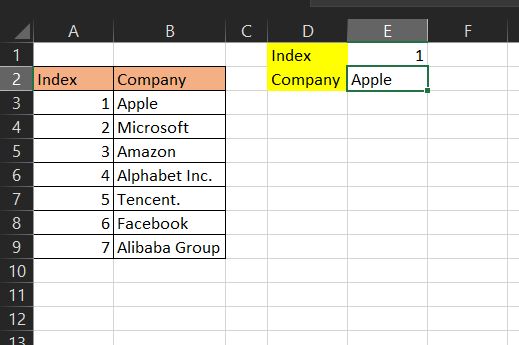Example 2: Here I want to pick a month and we want it to display the sum of revenue for that month picked.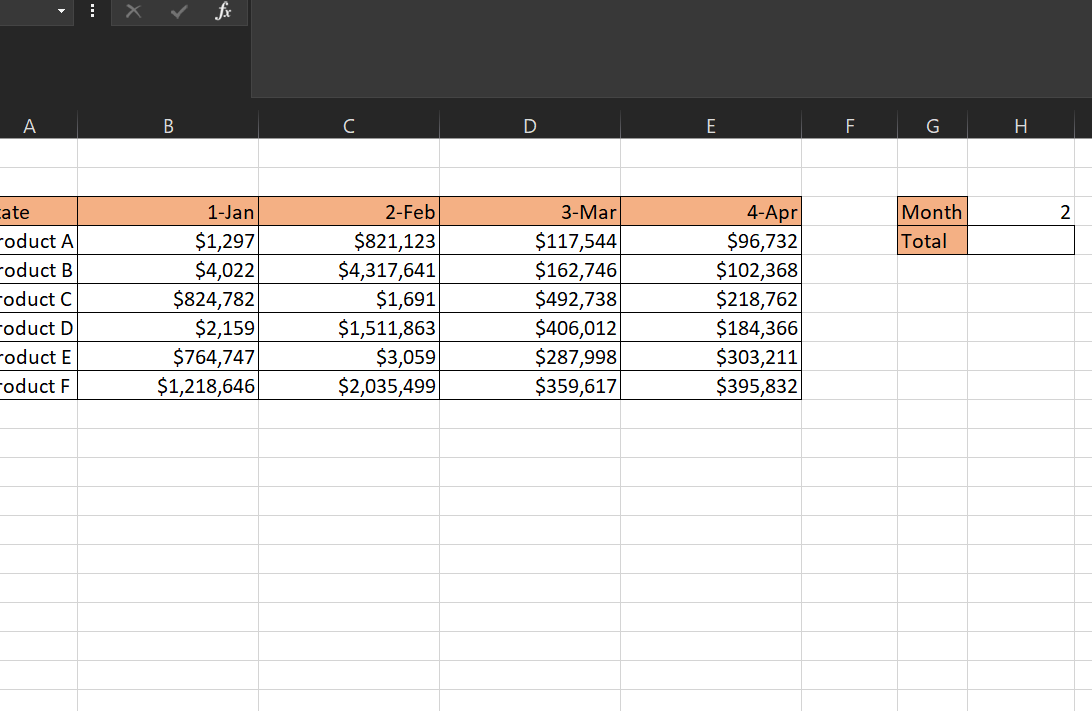Now, if we want to get the sum of the month picked in H4. Let us follow the next step

Step 2: In H3, we would put a number 1, 2, or 3 which represents Jan, Feb, and March. Thus We will enter =SUM(CHOOSE(H3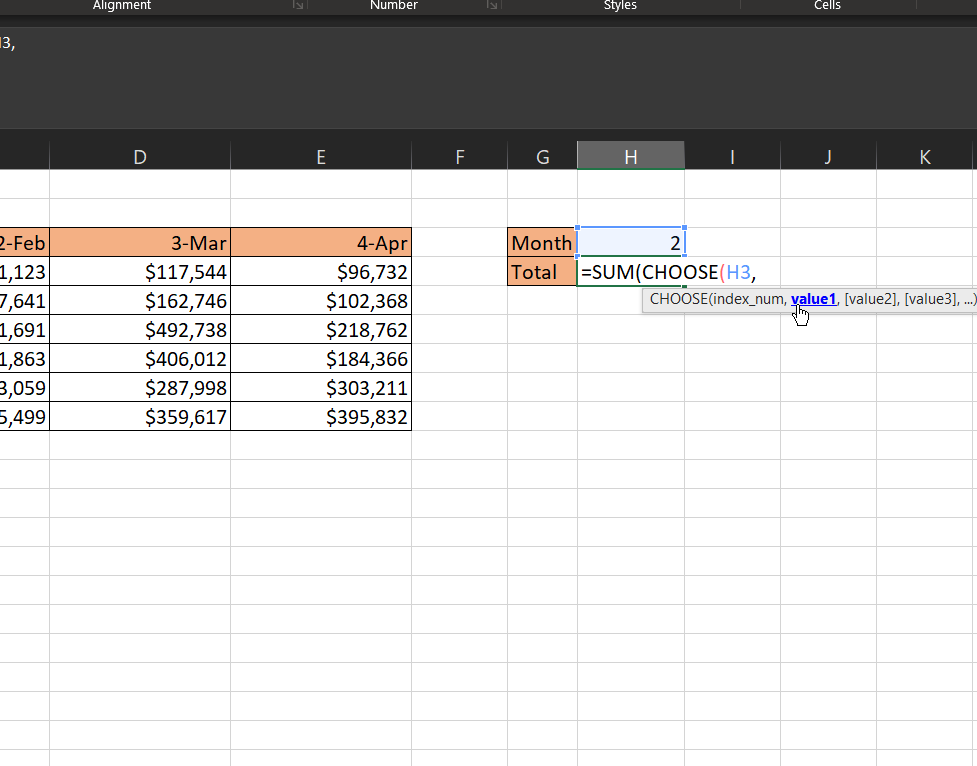Step 3: This will be followed by the values in each month. Thus This will be We will enter =SUM(CHOOSE(H3,B4:B9,C4:C9,D4:D9,E4:E9)) in H4 cell.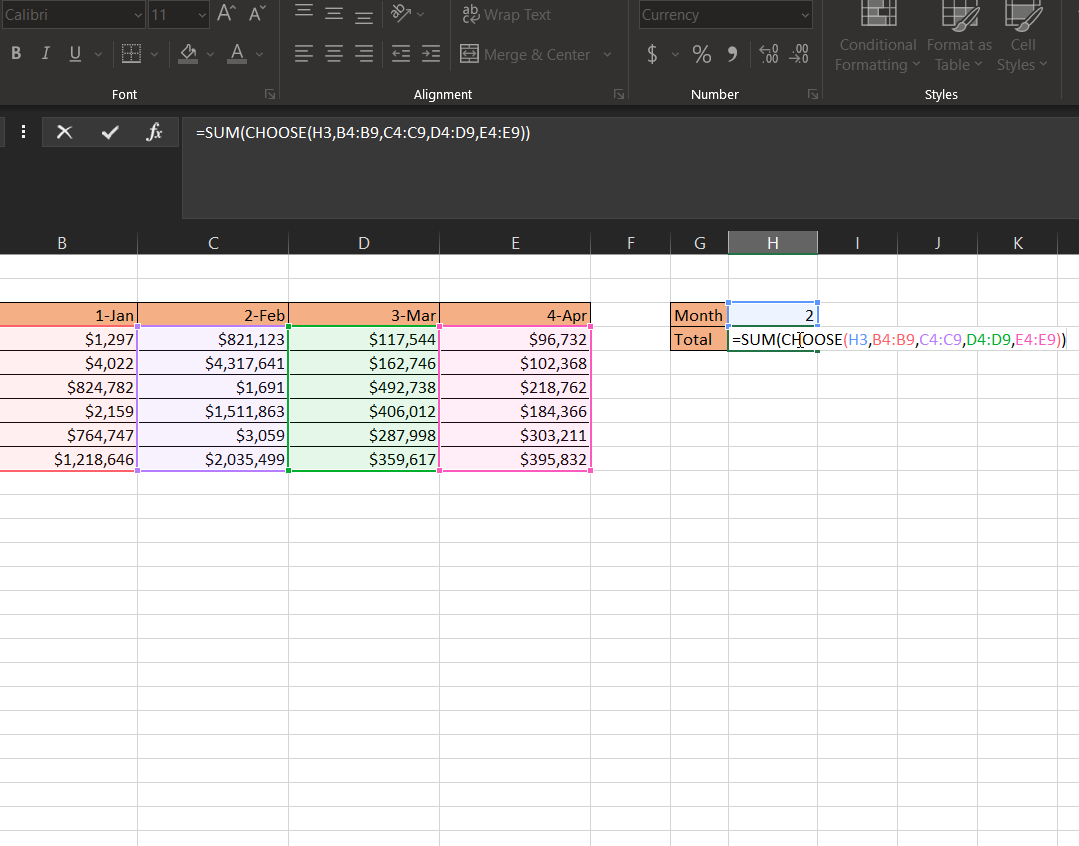Step 4: then we press ENTER on our keyboard. This will return the sum of values for 1 which is Jan.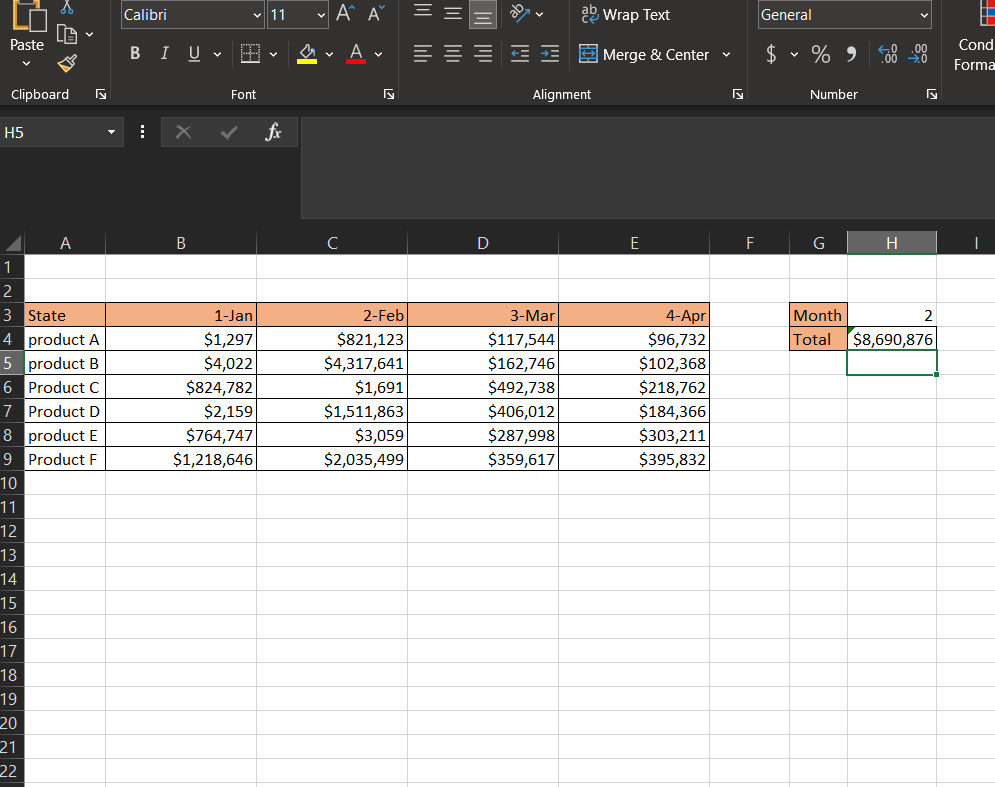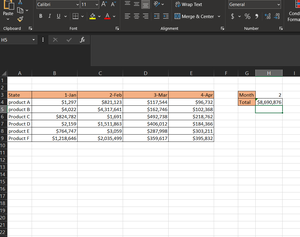My Personal Notes arrow_drop_up